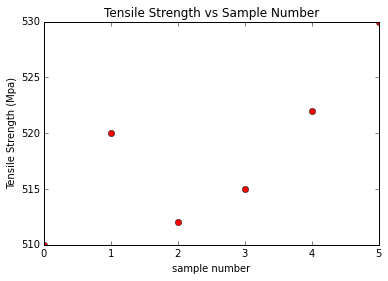# Chapter 9:Mechanical Properties of Metals¶

## Example 9.1 Page 310¶

In :
#initiation of variable
from math import acos, asin, sqrt, cos, sin
E=110.0*10**3  #Young's modulus of Copper in MPa
sigma=276.0   #Applied stress in MPa
l_o=305.0     #Original length in mm

#calculation
del_l=sigma*l_o/E #Deformation

#result
print" Elongation obtained is %.2f mm \n" %del_l

 Elongation obtained is 0.77 mm



## Example 9.2 Page 312¶

In :
#initiation of variable
from math import acos, asin, sqrt, cos, sin, pi
del_d=-2.5*10**-3 #Deformation in diameter in mm
d_0=10.0   #Initial diameter in mm
v=0.34 #Poisson ratio for brass
E=97*10**3 #Modulus of elasticity in MPa

#calculation
e_x=del_d/d_0 # Strain in x-direction
e_z=-e_x/v
sigma=e_z*E      # Stress produced
F=sigma*pi*(d_0**2)/4

#result
print" Strain in x-direction is %.1e" %e_x
print" Strain in z-direction is %.2e" %e_z
print" Applied force is %d N" %F
print " Answer in book is 5600N. It is due to rounding off at intermediate steps"

 Strain in x-direction is -2.5e-04
Strain in z-direction is 7.35e-04
Applied force is 5601 N
Answer in book is 5600N. It is due to rounding off at intermediate steps


## Example 9.3 Page 316¶

In :
#initiation of variable
from math import acos, asin, sqrt, cos, sin, pi, floor
sigma_2=150.0 # Stress at a point in MPa
sigma_1=0.0 # Stress at a point in MPa
epsilon_2=0.0016 # Strain at a point in MPa
epsilon_1=0.0 # Strain at a point in MPa
d_0=12.8*10**-3 #Initial Diameter in m
sigma=450.0*10**6     #tensile strength in MPa
l0=250.0;     #Initial length in mm
e=0.06;     #strain

# Part A
#calculation
E=(sigma_2-sigma_1)/(epsilon_2-epsilon_1) #Young's Modulus = stress/strain

#result
print " Modulus of elasticity is %.1f GPa  which is very close to its true value 97 GPa" %(E/10**3)

# Part C
A_0=pi*d_0**2/4.0
F=sigma*A_0
dl=e*l0

#result
print" Maximum load sustained is %d N" %(floor(F/10)*10)

#part D
print" Change in length is %d mm" %dl;

 Modulus of elasticity is 93.8 GPa  which is very close to its true value 97 GPa
Maximum load sustained is 57900 N
Change in length is 15 mm


## Example 9.4 Page 323¶

In :
#initiation of variable
from math import acos, asin, sqrt, cos, sin, pi, floor
di=12.8 #Initial diameter in mm
df=10.7 #Final diameter  in mm
sigma=460.0*10**6     #Tensile strength

# Part A
#calculation
RA = ((di**2-df**2)/di**2)*100 #Ductility in terms of Reduction Area

#result
print" Percent reduction in area is %d" %RA

# Part B
A_o=pi*di**2*10**-6/4.0
F=sigma*A_o
Af=pi*df**2/4
sigma_t=F/Af

#result
print" True stress at failure is %d MPa" %sigma_t
print "Answer in book is 660. It is due to founding off at intermediate stage"

 Percent reduction in area is 30
True stress at failure is 658 MPa
Answer in book is 660. It is due to founding off at intermediate stage


## Example 9.5 Page 323¶

In :
#initiation of variable
from math import acos, asin, sqrt, cos, sin, pi, floor, log
sigma_t=415.0 #True stress in MPa
et=0.1 #True strain
K=1035.0 # Bulk modulus in MPa

#calculation
n=log(sigma_t/K)/log(et)

#result
print" Strain hardening coefficient is %.2f" %n;

 Strain hardening coefficient is 0.40


## Example 9.6 Page 332¶

In :
#initiation of variable
%pylab inline
import numpy as np
import matplotlib.pyplot as plt
from math import acos, asin, sqrt, cos, sin, pi, floor, log
n=[0.0, 1.0, 2.0, 3.0, 4.0, 5.0]
T_S=[510.0, 520.0, 512.0, 515.0, 522.0, 530]

#First and Last points are arbitrary to plot the required points

#partA
plt.plot(n,T_S,'ro')
plt.xlabel('sample number')
plt.ylabel('Tensile Strength (Mpa)')
plt.title('Tensile Strength vs Sample Number')
plt.show()

#Mean Tensile strength
i=2;
TS_mean=0;

for i in range (1,5):
TS_mean=TS_mean+(T_S[i]/4);

print " Average tensile strength is %d MPa." %TS_mean;
#Standard Deviation

#partB
j=0
std=0

for i in range (1,5):
std=std+((T_S[i]-TS_mean)**2/(4.0-1))

print" Standard deviation is %.1f MPa" %(sqrt(std))

Populating the interactive namespace from numpy and matplotlib

WARNING: pylab import has clobbered these variables: ['sin', 'std', 'cos', 'sqrt', 'log', 'pi', 'floor']
%matplotlib prevents importing * from pylab and numpyAverage tensile strength is 517 MPa.
Standard deviation is 4.6 MPa


## Example 9.7 (Design Problem 9.1) Page 334¶

In :
#initiation of variable
import numpy as np
import matplotlib.pyplot as plt
from math import acos, asin, sqrt, cos, sin, pi, floor, log
f = 220000.0 # Maximum load in N
sigma_y = 310.0 # Minimum field strength in MPa
sigma_t = 565.0 # Tensile strength strength in MPa
N= 5.0 # Factor of safety

#calculation
sigma_w = sigma_y*1.0e6/N
d = 2*sqrt (f/(2*pi*sigma_w))# Calculation of diameter

#result
print" Diameter of each of two rods should be %.1f mm" %(d*1e3)

 Diameter of each of two rods should be 47.5 mm